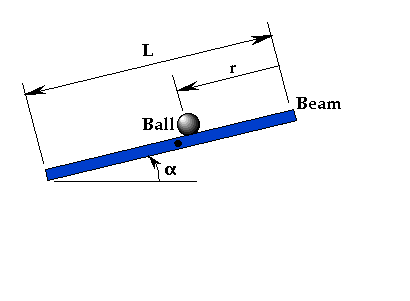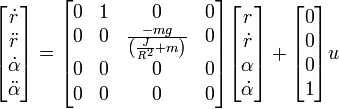# Ball and beam system

Lars Cremean, 29 September 2003

{{{2}}}

## System Description

A ball is placed on a beam, see figure below, where it is allowed to roll with 1 degree of freedom along the length of the beam. A motor is connected to the beam at the center, and produces a torque that causes the beam to rotate. When the angle is changed from the horizontal position, gravity causes the ball to roll along the beam. A controller will be designed for this system so that the ball's position can be manipulated.For this problem, we will assume that the ball rolls without slipping and friction between the beam and ball is negligible. The constants and variables for this example are defined as follows:

 M mass of the ball 0.11 kg R radius of the ball 0.015 m g gravitational acceleration 9.8 m/s^2 J ball's moment of inertia 9.99e-6 kgm^2 r ball position coordinate alpha beam angle coordinate

A state space representation of the system can be found to beThis representation is included in the Simulink model provided, along with full-state (i.e. all of the state variables are available to the controller) feedback control with reference input.

### Instructions

Right-click {{{2}}} and save it to a local drive. Open it with MATLAB (Simulink) and follow the instructions given above.